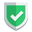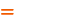我们相信：世界是美好的，你是我也是。平行空间的世界里面，不同版本的生活也在继续...

## `nparray`基本概念

``````import numpy as np
aa = np.array([1, 2])
a2 = np.random.randint(1, 3, size=(2, 3))
print(aa.shape)
print(a2.shape)``````

## `.expand_dims()`的`axis`参数

`.expand_dims()`是个扩维函数，第一个参数就是被扩维的数组，第二个参数`axis`就是被扩容的位置。为了更好的理解这个函数，最好先观察它的维度信息。

``````import numpy as np
aa = np.array([1, 2])
print(aa.shape,aa,aa)
a2 = np.expand_dims(aa,axis=0)
print(a2.shape,a2[0,1],a2,a2)
a3 = np.expand_dims(a2,axis=1)
print(a3.shape,a3[0,0,1],a3,a3)``````

`axis`取负数的时候，就是从最后的位置添加的。`-1`就是添加在最后，`-2`就是添加在倒数第二位，后面的向右移动。

``````a4 = np.expand_dims(a3,axis=-1)
print(a4.shape,a4[0,0,1,0],a4)
a5 = np.expand_dims(a4,axis=-2)
print(a5.shape,a5[0,0,1,0,0],a5)
a6 = np.expand_dims(a5,axis=-3)
print(a6.shape,a6[0,0,1,0,0,0],a6)``````

1(2,)``0
2(1,2)`【0】`[0,1]1
3(1,1,2)`【0】`[0,0,1]-1
4(1,1,2,1)`【0】`[0,0,1,0]-2
5(1,1,2,1,1)`【0】`[0,0,1,0,0]前四状态，第五动作，这里没动作

``numpy.AxisError: axis 2 is out of bounds for array of dimension 2``

## `.expand_dims()`扩维前后

``````import numpy as np
b1 = np.random.randint(1, 3, size=(2, 3))
print(b1)
# [[2 2 2]
#  [1 1 2]]
b2 = np.expand_dims(b1, axis=0)
b1=100
print(b1)
# [[100   1   1]
#  [  2   1   2]]
print(b2)
# [[[100   2   2]
#   [  1   1   2]]]``````

## 综述如果本文对您有帮助，或者节约了您的时间，欢迎打赏瓶饮料，建立下友谊关系。本博客不欢迎：各种镜像采集行为。请尊重原创文章内容，转载请保留作者链接。【福利】 腾讯云最新爆款活动！1核2G云服务器首年50元！【源码】本文代码片段及相关软件，请点此获取更多信息【绝密】秘籍文章入口，仅传授于有缘之人python    numpy